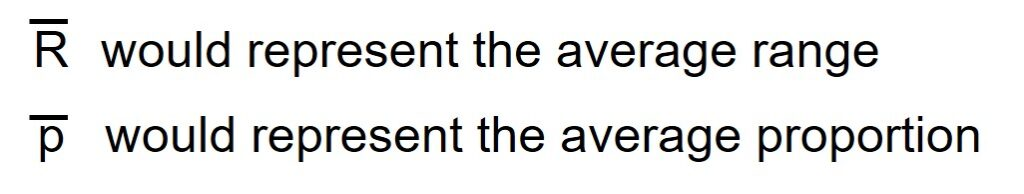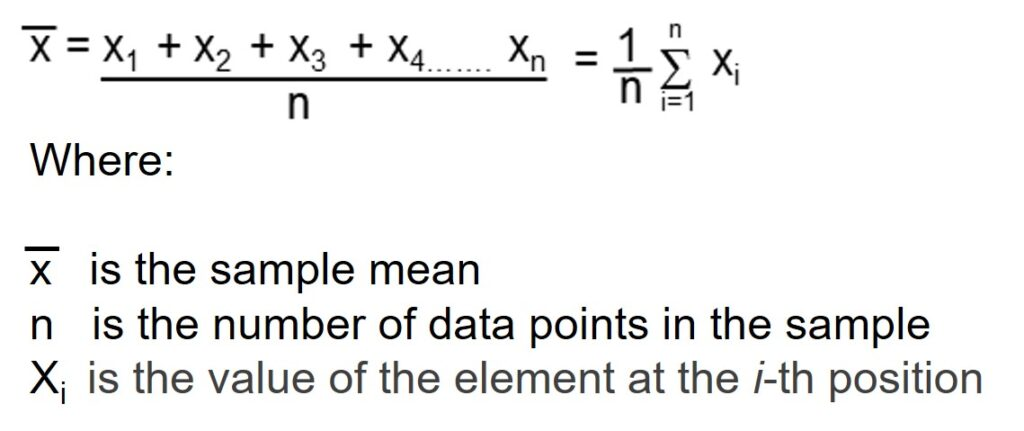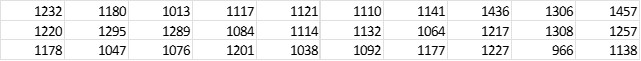There are three measures of central tendency for data. One is the mean or x-bar, the others are the median and mode. Let’s take a closer look at the mean or x-bar.

## Overview: What is x-bar?

In statistics, the Latin small letter x is used to represent a variable in a set of sample data. A horizontal bar over a letter indicates the letter represents the average or mean of whatever the variable represents. For example:To calculate the x-bar or sample mean, you would sum all the data points in the sample and then divide by the number of data points. This will be represented by the following formula:But there is a caveat for using the x-bar as a measure of central tendency. Beware that the average of a data set can be skewed by an outlier on the high or low side.

## An Industry Example of x-bar

The Manufacturing Supervisor wanted to know the average pieces produced per day on his machine. He asked the local Six Sigma Green Belt to help collect the data and do the calculations. Below is the sample data for 30 days of production and the calculation for the x-bar or average number of pieces produced per day. It is just a matter of adding up the 30 daily data points and dividing by the number of data points which was 30.Mean or x-bar = 1174 pieces

### What does the x-bar measure in a data set?

The x-bar, also known as the arithmetic mean or average, is a measure of the mathematical center of sample data and will represent the central tendency of that data.

### What is the difference between the x-bar and median of a set of sample data?

The x-bar is the calculated center of the data while the median represents the physical center of the data.

### Is the x-bar a good measure of central tendency for my sample data?

The x-bar is the most common measure of the central tendency of sample data. But be aware that the average of a set of data can be skewed if there are unusually high or low values in the data.